NEET  >  NEET Previous Year Questions (2014-22): Mechanical Properties of Fluids

# NEET Previous Year Questions (2014-22): Mechanical Properties of Fluids - Notes | Study Physics Class 11 - NEET

 1 Crore+ students have signed up on EduRev. Have you?

Q.1. A spherical ball is dropped in a long column of a highly viscous liquid. The curve in the graph shown, which represents the speed of the ball (v) as a function of time (t) is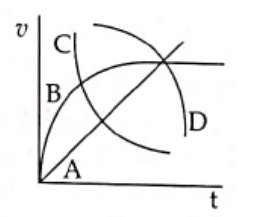(1) B
(2) C
(3) D
(4) A
Ans: (1)
Solution: Initial speed of ball is zero and it finally attains terminal speed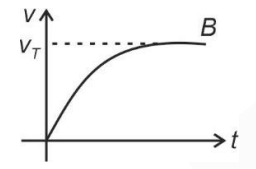Q.2. If a soap bubble expands, the pressure inside the bubble
(1) Increases
(2) Remains the same
(3) Is equal to the atmospheric pressure
(4) Decreases              

Ans: (4)
Solution:
Excess pressure inside the bubble =
P = P+ 4T/R
as ‘R’ increases ‘P’ decreases

Q.2. The velocity of a small ball of mass M and density d, when dropped in a container filled with glycerine becomes constant after some time. If the density of glycerine is d/2, then the viscous force acting on the ball will be:      
A: 3/2Mg
B: 2Mg
C: Mg/2
D: Mg
Ans: C
Solution:
Mass = MDensity of ball = d
Density of glycerine = d/2Q.3. A soap bubble, having radius of 1 mm, is blown from a detergent solution having a surface tension of 2.5 × 10-2 N/m. The pressure inside the bubble equals at a point Z0 below the free surface of water in a container. Taking g = 10 m/s2, density of water = 103 kg/m3, the value of Z0 is     
A: 100 cm
B: 10 cm
C: 1 cm
D: 0.5 cm
Ans: C
Solution:
Excess pressure = 4T/R, Gauge pressure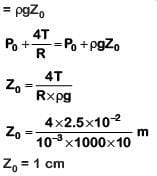Q.4. A small hole with area area of cross-section 2 mm2 is present near the bottom of a fully filled open tank of height 2 m. Taking g = 10 m/s2, the rate of flow of water through the open hole would be nearly    
A: 12.6 × 10–6 m3/s
B: 8.9 × 10–6 m3/s
C: 2.23 × 10–6 m3/s
D: 6.4 × 10–6 m3/s
Ans: A
Solution: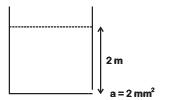Rate of liquid flow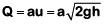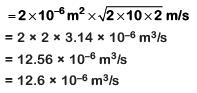Q.5. A small sphere of radius 'r' falls from rest in a viscous liquid. As a result, heat is produced due to viscous force. The rate of production of heat when the sphere attains its terminal velocity, is proportional to:-    
A: r3
B: r2
C: r5
D: r4
Ans: C
Solution: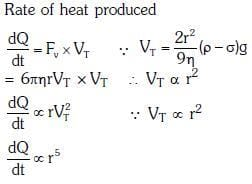Q 5. A U tube with both ends open to the atmosphere, is partially filled with water. Oil, which is immiscible with water, is poured into one side until it stands at a distance of 10 mm above the water level on the other side. Meanwhile the water rises by 65 mm from its original level (see diagram). The density of the oil is:-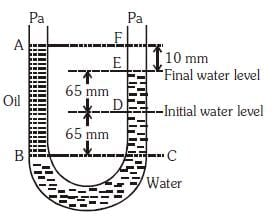A: 425 kg m–3
B: 800 kg m–3
C: 928 kg m–3
D: 650 kg m–3
Ans:
C
Solution: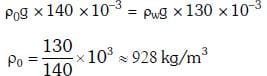Q 7. Two non-mixing liquids of densities ρ and nρ (n > 1) are put in container. The height of each liquid is h. A solid cylinder of length L and density d is put in this container. The cylinder floats with its axis vertical and length pL (p < 1) in the denser liquid. The density d is equal to    
A: {1 + (n - 1)p}ρ
B: {1 + (n + 1)p}ρ
C: {2+(n + 1)p}ρ
D: {2 + (n - 1)p}ρ
Ans:
A
Solution: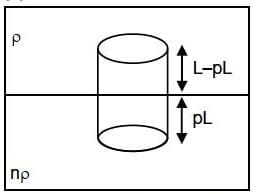wt of body = upthrust by the two liquids
If A = Area of section then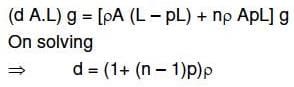Q 8. The approximate depth of an ocean is 2700 m. The compressibility of water is 45.4 x 10-11 Pa-1 and density of water is 103 kg/m3. What fractional compression of water will be obtained at the bottom of the ocean?    
A: 1.4 x 10-2
B: 0.8 x 10-2
C: 1.0 x 10-2
D: 1.2 x 10-2
Ans:
D
Solution: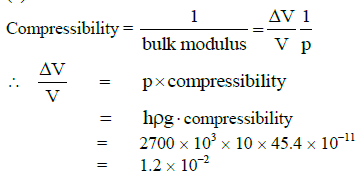Q 9. A wind with speed 40 m/s blows parallel to the roof of a house. The area of the roof is 250 m2. Assuming that the pressure inside the house is atmospheric pressure, the force exerted by the wind on the roof and the direction of the force will be:
(Pair = 1.2 kg/m3)    
A: 2.4 x 105 N, downwards
B: 4.8 x 105 N, downwards
C: 4.8 x 105 N,upwards
D: 2.4 x 105 N, upwards
Ans:
D
Solution: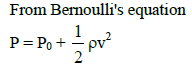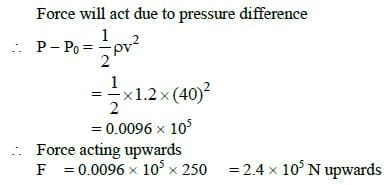Q 10. A certain number of spherical drops of a liquid of radius ‘r’ coalesce to form a single drop of radius ‘R’ and volume ‘V’. If ‘T’ is the surface tension of the liquid, then:    
A: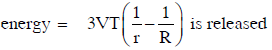B: energy is neither released nor absorbed
C: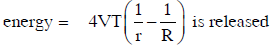D: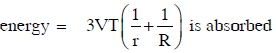Ans:
A
Solution: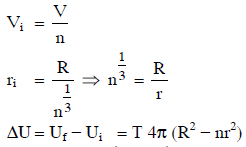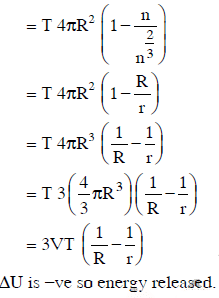The document NEET Previous Year Questions (2014-22): Mechanical Properties of Fluids - Notes | Study Physics Class 11 - NEET is a part of the NEET Course Physics Class 11.
All you need of NEET at this link: NEET

## Physics Class 11

127 videos|464 docs|210 tests
 Use Code STAYHOME200 and get INR 200 additional OFF

## Physics Class 11

127 videos|464 docs|210 tests

### How to Prepare for NEET

Read our guide to prepare for NEET which is created by Toppers & the best Teachers

Track your progress, build streaks, highlight & save important lessons and more!

,

,

,

,

,

,

,

,

,

,

,

,

,

,

,

,

,

,

,

,

,

;